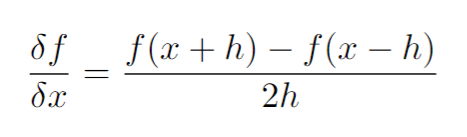# Avoiding loop for sequential update on tensors

I have a tensor `a` with shape (100,140,140) which is actually a batch (size 100) of matrices of size (140x140). You can assume these matrices are actually vectors with 140x140 = 19600 components.

I also have a function `myfunc(a, b, c, d)` where b, c, d are some constant tensors. `myfunc()` outputs a batch of scalers. Now I want to find the gradient of `myfunc(a, b, c, d)` with respect to the vectors in tensor `a` using Finite Difference Method:What I am trying to do right now is something like this:

``````grad_a = torch.empty_like(a)

for i in range(a.shape):
for j in range(a.shape):
a[:,i,j] += h    #h is the step size
out1 = myfunc(a, b, c, d)
a[:,i,j] -= 2*h
out2 = myfunc(a, b, c, d)